# Virtual COMSATS Inferential Statistics Lecture4 Ossam Chohan Assistant

• Slides: 18Virtual COMSATS Inferential Statistics Lecture-4 Ossam Chohan Assistant Professor CIIT Abbottabad 1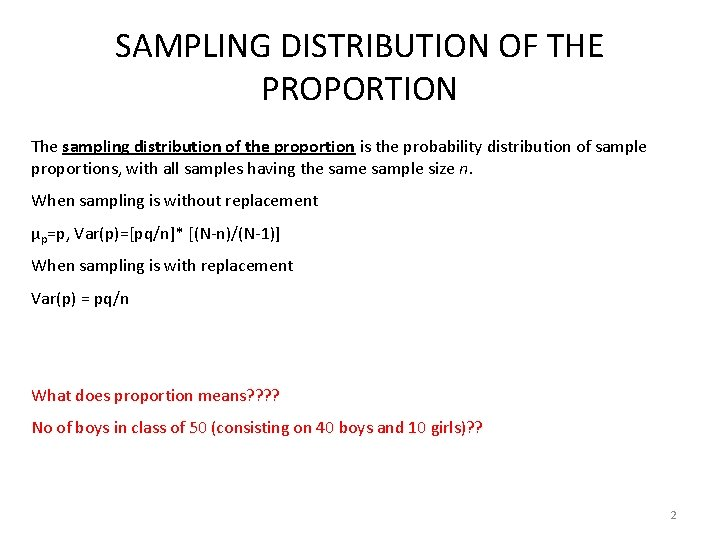SAMPLING DISTRIBUTION OF THE PROPORTION The sampling distribution of the proportion is the probability distribution of sample proportions, with all samples having the sample size n. When sampling is without replacement µp=p, Var(p)=[pq/n]* [(N-n)/(N-1)] When sampling is with replacement Var(p) = pq/n What does proportion means? ? No of boys in class of 50 (consisting on 40 boys and 10 girls)? ? 2Example-4 Sampling with proportions • A population consists of N=6 values 1, 3, 6, 8, 9 and 12. Draw all possible samples of size 3 (WOR). Find the proportion of even numbers and construct sampling distribution of sample proportions and verify the results. 3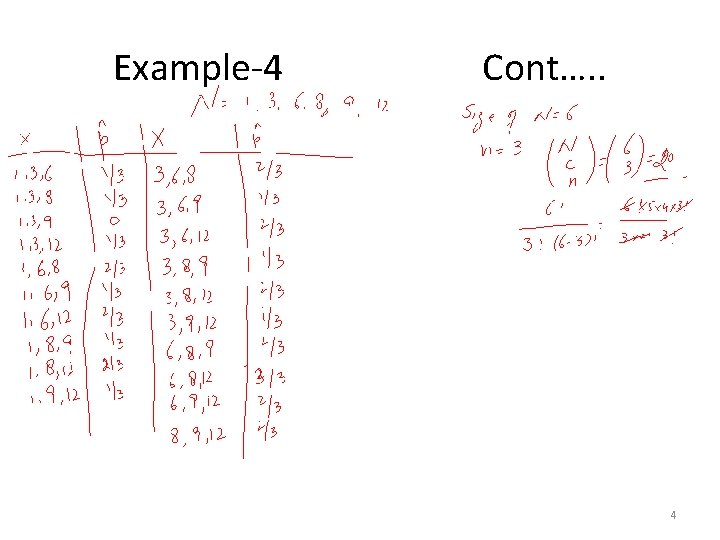Example-4 Cont…. . 4Example-4 cont… 5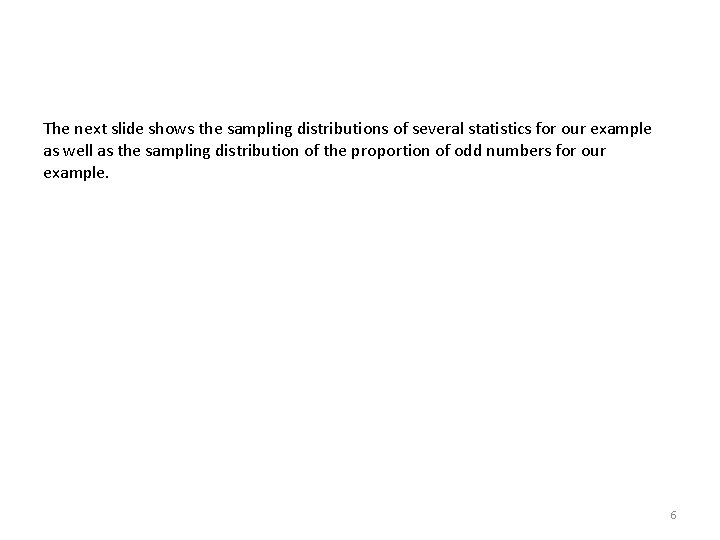The next slide shows the sampling distributions of several statistics for our example as well as the sampling distribution of the proportion of odd numbers for our example. 6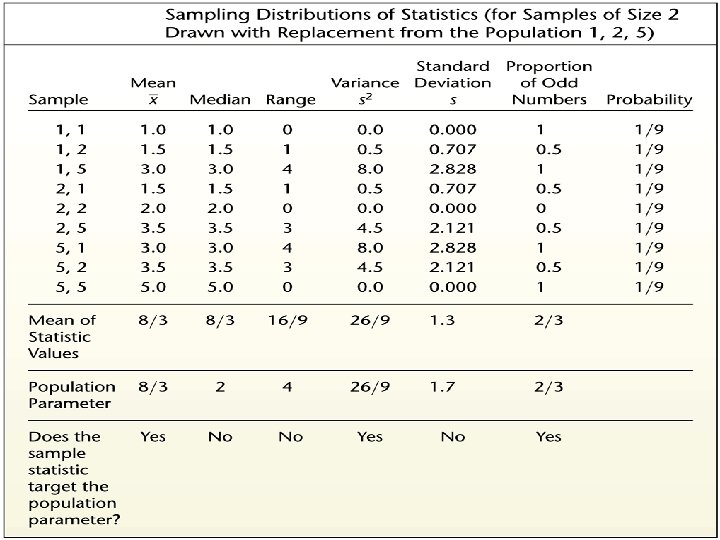7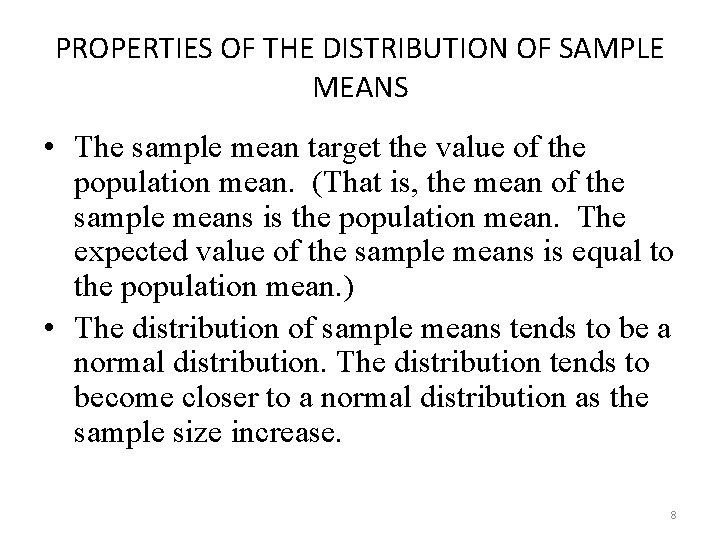PROPERTIES OF THE DISTRIBUTION OF SAMPLE MEANS • The sample mean target the value of the population mean. (That is, the mean of the sample means is the population mean. The expected value of the sample means is equal to the population mean. ) • The distribution of sample means tends to be a normal distribution. The distribution tends to become closer to a normal distribution as the sample size increase. 8PROPERTIES OF THE DISTRIBUTION OF SAMPLE PROPORTIONS • The sample proportions tend to target the value of the population proportion. (That is, the mean of the sample proportions is the population proportion. The expected value of the sample proportion is equal to the population proportion. ) • The distribution of sample proportions tends to be a normal distribution. 9Example-5 (Difference between means) • Suppose N 1=4, 6, 8 and n 1=2 • Suppose N 2=1, 2, 3 and n 2=2 • Find all possible differences between the sample means of two populations. • Construct the sampling distribution of and computer mean and variances. • Verify the results 10111213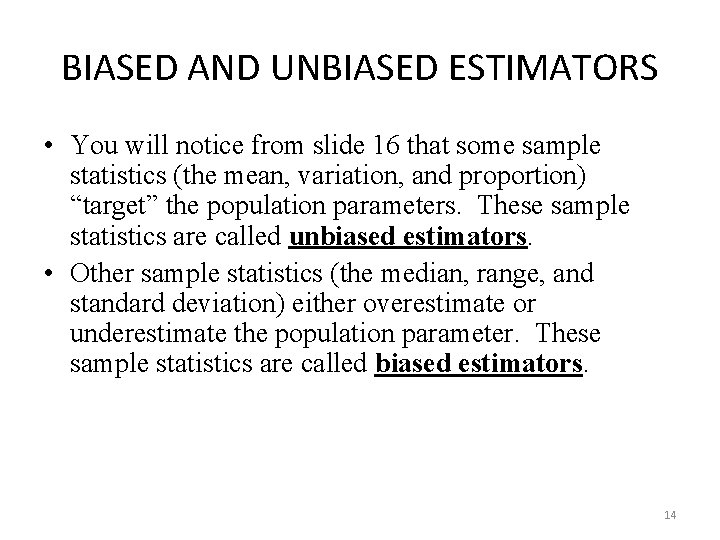BIASED AND UNBIASED ESTIMATORS • You will notice from slide 16 that some sample statistics (the mean, variation, and proportion) “target” the population parameters. These sample statistics are called unbiased estimators. • Other sample statistics (the median, range, and standard deviation) either overestimate or underestimate the population parameter. These sample statistics are called biased estimators. 14A COMMENT ON THE STANDARD DEVIATION Even though the standard deviation is biased, the bias is relatively small in a large sample. As a result s is often used to estimate σ. This is an important property and we will discuss it in details in estimation section. 15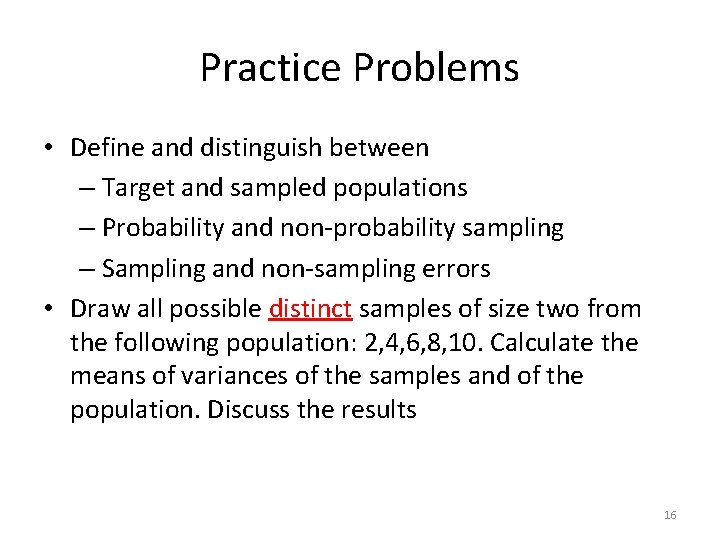Practice Problems • Define and distinguish between – Target and sampled populations – Probability and non-probability sampling – Sampling and non-sampling errors • Draw all possible distinct samples of size two from the following population: 2, 4, 6, 8, 10. Calculate the means of variances of the samples and of the population. Discuss the results 16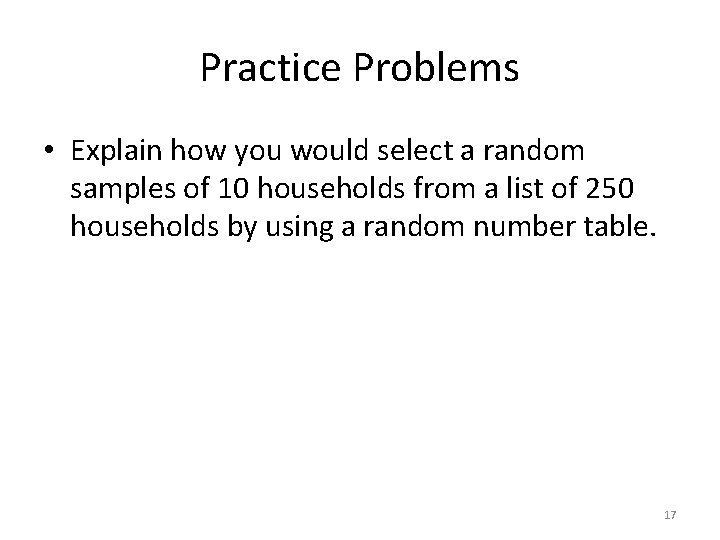Practice Problems • Explain how you would select a random samples of 10 households from a list of 250 households by using a random number table. 17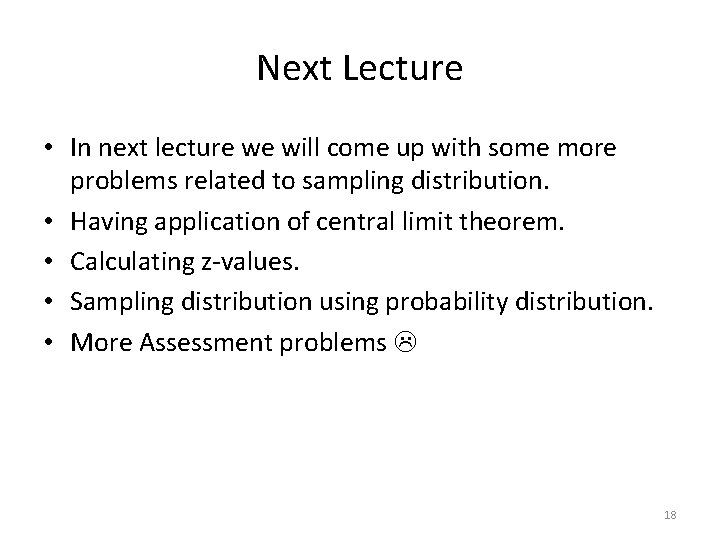Next Lecture • In next lecture we will come up with some more problems related to sampling distribution. • Having application of central limit theorem. • Calculating z-values. • Sampling distribution using probability distribution. • More Assessment problems 18